Worksheets and No Prep Teaching Resources Math Angles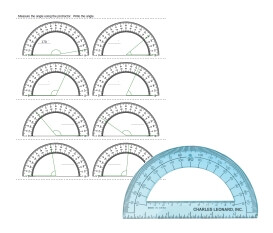Protractor Printables, Worksheets, and Lessons

Measure the Angle Using the Protractor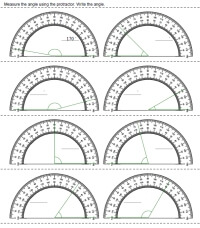Protractor on page - first answer given (degrees are multiples of 5 only) Protractor on page (degrees are multiples of 5 only) Protractor on page - first answer given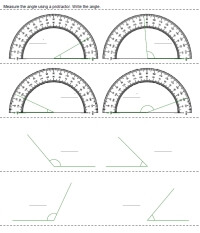Protractor on page for half of problems - first answer given (degrees are multiples of 5 only) Protractor on page for half of problems (degrees are multiples of 5 only) Protractor on page for half of problems - first answer given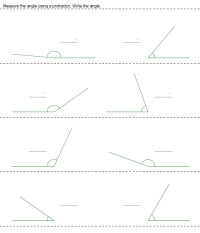Using own protractor - first answer given (degrees are multiples of 5 only) Using own protractor (degrees are multiples of 5 only) Using own protractor - first answer given

Draw an Angle Using a Protractor
Protractor on page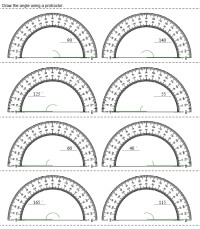Protractor on page - semicircle shown for angle - first answer given (degrees are multiples of 5 only) Protractor on page - semicircle shown for angle (degrees are multiples of 5 only) Protractor on page - semicircle shown for angle - first answer given Protractor on page - first answer given (degrees are multiples of 5 only) Protractor on page (degrees are multiples of 5 only) Protractor on page - first answer given

Protractor Drawn for Half of Problems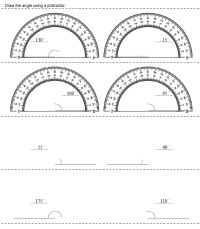Semicircle shown for angle - first answer given (degrees are multiples of 5 only) Semicircle shown for angle (degrees are multiples of 5 only) Semicircle shown for angle - first answer given First answer given (degrees are multiples of 5 only) Protractor on page (degrees are multiples of 5 only) First answer given

Need to Use your Own Protractor to Draw an Angle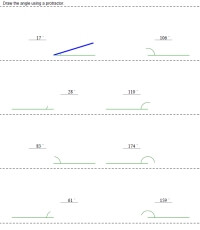Semicircle shown for angle - first answer given (degrees are multiples of 5 only) Semicircle shown for angle (degrees are multiples of 5 only) Semicircle shown for angle - first answer given First answer given (degrees are multiples of 5 only) Protractor on page (degrees are multiples of 5 only) First answer given

Measure of Angles - Reading a Protractor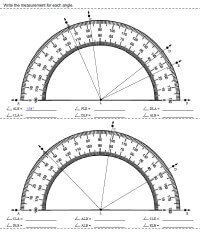Easier Angles: Reading the protractor - Multiples of 10 degrees only Easier Angles: Reading the protractor - Multiples of 5 degrees only Easier Angles: Reading the protractor

With Addition or Subtraction - Measure of Angles - Reading a Protractor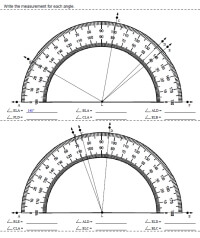Most are easier angles - one or two requires addition or subtraction: Reading the protractor - Multiples of 10 degrees only Most are easier angles - one or two requires addition or subtraction: Reading the protractor - Multiples of 5 degrees only Most are easier angles - one or two requires addition or subtraction: Reading the protractor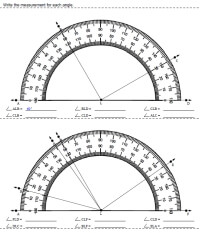More Challenging - Reading the protractor - Multiples of 10 degrees only More Challenging - Reading the protractor - Multiples of 5 degrees only More Challenging - Reading the protractor

Redraw a Triangle Using a Protractor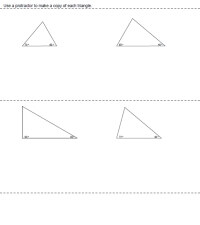Make a copy of a triangle using a protractor

Have a suggestion or would like to leave feedback?